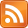Some subgroups of a group $G$ are normal, and some are not. It's not easy to get an intuition for what that means. A week or two after encountering the concept in my abstract algebra class, I knew the following:

• Normal subgroups $N$ of $G$ are the only ones you can quotient by; i.e. you can write $G/N$ and it's a valid group.
• They are the kernel of some homomorphism $G \rightarrow H$, where $H$ is any other group. Kernel means every element gets mapped to the identity (the subgroup is "killed").

But I didn't see any connection between these. I didn't get what the big idea behind normality, or even quotient groups, was supposed to be. I wanted to be able to answer questions by feeling: if I take this group and divide it by that, what should I get?

Here's a bit of intuition I eventually arrived at. Let's say you have a group of transformations -- say $GL(3)$, invertible linear transformations of 3-D space. These transformations might rotate stuff, grow/shrink it, or skew it. Each of these transformations has a determinant, a function from $GL(3) \rightarrow \mathbb{R}$ that tells you how much it changes the volume of a unit cube sitting at the origin.

Different transformations can have the same determinant; consider rotating the cube by 90° and rotating it by 180°. Neither of them change the volume, so both have determinant 1.

You might be interested in these transformations for how they move space around, not how they make it bigger or smaller. You're sick of everyone talking about determinants and wish they would just go away. If so, you can say "Okay, let's just hold the determinant constant at 1." Then you are looking at a perfectly nice subgroup -- $SL(3)$, the normal subgroup of all matrices with determinant 1.

It's normal because it is the kernel of the determinant (which is a homomorphism). Intuitively, you got it by finding the dial labeled 'DETERMINANT', holding it steady, then watching the resulting group.

Now flip everything around and suppose that, actually, you don't care about the distinction between rotating 90° and rotating 180°. They both have determinant 1, so you wish they would be treated as the same object. In Dan Piponi's words, you can wear a certain kind of filter over your eyes that erases that distinction -- instead of $GL(3)$, form the group $GL(3)/SL(3)$ and look at that instead.

The '$/$' symbol can be read as "ignoring the distinctions of" -- so if you look at $GL(3)$, but ignore the distinctions between elements of $SL(3)$, what do you get? Well, you're ignoring every aspect of your transformations except for how they scale things. Scaling has a pretty simple group structure -- scaling by 2 is inverted by scaling by $\frac{1}{2}$, etc. It's just $\mathbb{R}^\times$, the group of real numbers under multiplication.

So $GL(3)/SL(3) \,\simeq\, \mathbb{R}^\times$, which is a fact.

Another way to see this is to think again of finding the dial labeled 'DETERMINANT' and only watching it. The group you get when you do this is going to be made out of the values that the determinant takes on -- i.e. $\mathbb{R}$. So, if you have a homomorphism $\psi: G \rightarrow H$ that kills some normal subgroup $N$, then $G / N \,\simeq\, H$. That's the first isomorphism theorem.

More examples (ripped from Wikipedia, where you go to see everything that surprises you, rendered dispassionately in black-and-white text):

• $\mathbb{Z}/3\mathbb{Z} \,\simeq\, \mathbb{Z}_3$, because if I'm in the integers but ignore the distinctions between different multiples of three, being at $5$ looks just like being at $2$. The homomorphism here is "mod by 3".
• $\mathbb{R}/\mathbb{Z} \,\simeq\, C_\infty$, the circle group, because if I'm in the reals but ignore the distinctions between different integers, being at $1.03$ looks just like being at $1908.03$. The homomorphism here is "chop off the integer part".
• $(\mathbb{Z}_2 \times \mathbb{Z}_2) / \mathbb{Z}_2 \,\simeq\, \mathbb{Z}_2$, because (thinking of $\mathbb{Z}_2 \times \mathbb{Z}_2$ as ordered pairs over $\{0, 1\}$) if I ignore what the left side is doing, the only distinction left to make is whether the right side is 0 or 1. The homomorphism here sends $(a, b) \rightarrow b$.

And since the first isomorphism theorem also holds for rings, we can do this one. Define $\mathbb{Q}[x]$ to be the set of all polynomials with rational coefficients, and $\langle x^2 - 3 \rangle$ to be the subset of these polynomials that are multiples of $x^2 - 3$. Then:

• $\mathbb{Q}[x]/\langle x^2 - 3 \rangle \,\simeq\, \mathbb{Q}[\sqrt{3}]$, all numbers of the form $a + b\sqrt{3}$. The homomorphism here is "take your polynomial and plug in $\sqrt{3}$ for $x$". It sends all multiples of $x^2 - 3$ to $0$ and anything else to some element of $\mathbb{Q}[\sqrt{3}]$.

Many thanks to Tim Gowers' blog!